[BACK]
 Computers, Materials & ContinuaDOI:10.32604/cmc.2022.031909Article

Intelligent Optimization-Based Clustering with Encryption Technique for Internet of Drones Environment

1Department of Information Technology, College of Computer and Information Sciences, Princess Nourah bint Abdulrahman University, P.O. Box 84428, Riyadh 11671, Saudi Arabia
2Department of Industrial Engineering, College of Engineering at Alqunfudah, Umm Al-Qura University, Saudi Arabia
3Department of Computer Science, College of Science & Art at Mahayil, King Khalid University, Saudi Arabia
4Faculty of Computers and Information, Computer Science Department, Menoufia University, Egypt
5Department of Digital Media, Faculty of Computers and Information Technology, Future University in Egypt, New Cairo 11845, Egypt
6Department of Computer and Self Development, Preparatory Year Deanship, Prince Sattam bin Abdulaziz University, AlKharj, Saudi Arabia
*Corresponding Author: Anwer Mustafa Hilal. Email: A.hilal@psau.edu.sa
Received: 29 April 2022; Accepted: 17 June 2022

Abstract: The recent technological developments have revolutionized the functioning of Wireless Sensor Network (WSN)-based industries with the development of Internet of Things (IoT). Internet of Drones (IoD) is a division under IoT and is utilized for communication amongst drones. While drones are naturally mobile, it undergoes frequent topological changes. Such alterations in the topology cause route election, stability, and scalability problems in IoD. Encryption is considered as an effective method to transmit the images in IoD environment. The current study introduces an Atom Search Optimization based Clustering with Encryption Technique for Secure Internet of Drones (ASOCE-SIoD) environment. The key objective of the presented ASOCE-SIoD technique is to group the drones into clusters and encrypt the images captured by drones. The presented ASOCE-SIoD technique follows ASO-based Cluster Head (CH) and cluster construction technique. In addition, signcryption technique is also applied to effectually encrypt the images captured by drones in IoD environment. This process enables the secure transmission of images to the ground station. In order to validate the efficiency of the proposed ASOCE-SIoD technique, several experimental analyses were conducted and the outcomes were inspected under different aspects. The comprehensive comparative analysis results established the superiority of the proposed ASOCE-SIoD model over recent approaches.

Keywords: Internet of drones; atom search algorithm; clustering; encryption; signcryption

1  Introduction

Internet of Drones (IoD) is defined as a platform that is created to provide users with accessibility and control upon drones through internet . In general, drones can be quickly turned into easily-accessible devices. Every single user can perform distinct operations using multiple drones under controlled airspace. Even though technology helps in mass production of onboard elements of Unmanned Aerial Vehicles (UAVs) such as energy storage batteries, sensors, and processors, the execution boundaries of such components hinder and diminish the application of UAVs [2,3]. IoD is a concept of coupling the drones and vehicles together using cloud mobility operations in order to achieve distant drone control and access and smooth adaption of offloading with distant cloud storage abilities . UAVs with fixed wings have a primary advantage over UAVs with rotating wings i.e., less maintenance, low-cost repair and simple structure. These characteristics allow the customer to have more operating duration at minimum cost .

In general, drones have insufficient battery sources and their computational energy is confined to a certain level. These drawbacks affect the entire transmission efficacy in IoD. Routing process is mandatory for effective transmission and communication of data amongst drones . It is challenging to ensure effective transmission of data amongst drones due to quickly varying topology and the portability of drones from IoD. In this scenario, clustering, the hierarchal routing process is the only solution to overcome such issues. In a cluster, there exists both Cluster Members (CMs) and a Cluster Head (CH) which altogether form a network with sub groups . The selection of CH assumes a significant role in clustering and every CM is eligible to be an applicant in the selection of CH. The increasing familiarity of the drones has in turn inclined the occurrence of cyber-attacks toward UAV systems in the past few decades. A cyber attacker tend to focus on radio associations of UAV system so as to delay the ability of systems in terms of interacting with user devices . This inculcates the data required by the user’s mobile devices which controls Global Positioning System (GPS) signals . In general, image processing techniques are also linked with network atmospheres. Images are sensitive and intuitive in nature due to which the absence of appropriate protective measures may result in leakage of data like confidential information, security breach and loss of private information too . So, there is a need exists to prevent the increasing number of cyber-attacks that severely attack the privacy and security of the data, especially image information. In this background, image information can be saved through encryption processes.

Bera et al.  presented a Blockchain (BC)-based access control method for IoD environment which permits secure communication amongst the drones and between drones and Ground Station Server (GSS). The data collected by GSS procedure and individual communications are converted securely into blocks. Block is added at last from the BC, while the cloud services are associated with GSS using Ripple Protocol Consensus Algorithm (RPCA) from a peer-to-peer cloud server network. Wazid et al.  presented a novel light-weight user authentication method in which a user from IoD environment who requires data access from a drone directly is provided such access to the data in drone. Aftab et al.  presented a CH selection method based on connectivity with Base Station (BS) with Fitness Function (FF) that contains Residual Energy (RE) and information about the place of drones. Besides, route election can present an optimum path election based upon RE and the place of drones for effectual communications. Saif et al.  concentrated on performance evaluation of clustering technique by identifying wireless coverage service through increasing energy efficacy. The performance was evaluated through realistic model from ground to air channel Line-of-Sight (LoS). The outcomes depict that the CH efficiently connects the UAV and CMs at less energy expenditure. Bera et al.  presented and analyzed a novel BC-based secure structure for data management amongst IoD transmission entities. The presented method is capable of resisting numerous potential attacks that are important from Internet of Things (IoT)-allowed IoD environments.

The current study introduces Atom Search Optimization based Clustering with Encryption Technique for Secure Internet of Drones (ASOCE-SIoD) environment. The presented ASOCE-SIoD technique follows ASO-based Cluster Head (CH) and cluster construction technique. The presented model derives a FF involving multiple parameters especially trust parameters for secure process. In addition, signcryption technique is also applied to effectually encrypt the images captured by drones in IoD environment which enables secure transmission of images to the ground station. In order to validate the increased efficiency of the proposed ASOCE-SIoD model, numerous experimental analyses were conducted and the outcomes were examined under different aspects.

2  Design of ASOCE-SIoD Model

In this study, a novel ASOCE-SIoD approach has been developed to group the drones into clusters and encrypt the images captured by drones. The presented ASOCE-SIoD technique follows ASO-based CH and cluster construction technique. The presented model derives a FF involving multiple parameters especially trust parameters for secure process. In addition, signcryption technique is also applied to effectually encrypt the images captured by drones in IoD environment. Fig. 1 illustrates the overall processes involved in ASOCE-SioD method.Figure 1: Overall processes of the proposed method

2.1 Overview of ASO Algorithm

ASO is one of the recently-developed, physics-inspired, population-based heuristic approach that stimulates the atomic motion under control of constraint and interaction forces to project a better searching method for global optimization problems . The overall interaction forces to act upon i-th atom in d dimensional vector, i.e., the amount of repulsions and the attraction applied in vigorous modification of neighbor atom on i-th atom is as follows.

Fid(t)=jKbestrandjFijd(t)(1)

In Eq. (1), the random numbers with 0 and 1 are denoted via randj and Kbest which indicate a set of atom populations that comprises of initial K atoms and an optimal FF. Thus, the values of K need to be reduced in a linear fashion at iteration, using the following equation,

K(t)=N(N2)×tT(2)

In Eq. (2), the overall number of atoms in the atomic system is denoted via N, t indicates the existing iteration and T denotes the maximal amount of iterations. Fijd in (1) indicates the interaction force that j-th optimal atom exerted on i-th atom in d dimensional vector.

Fijd=η(t)[2(hij(t))13(hij(t))7]rijrij(3)

In Eq. (3), η(t) indicates the depth function for adjusting attractive or repulsion areas whereas hij(t)=rij/σ(t) indicates the ratio of distance between two atoms to the scaling length that is named as scaling distance between two atoms. r=xx denotes the location variance vector in which x=(xj1,xj2,xj3) indicates the location vector of j-th atom and x=(xi1,xi2,xi3) signifies the location vector of i-th atom. Therefore, rij implies the Euclidian distance between i-th and j-th atoms

rij=xjxi=(xj1xi1)2+(xj2xi2)2+(xj3xi3)2(4)

In (3), the depth function is described as follows.

η(t)=α(1t1T)3e20tT(5)

Here α indicates the depth weight and is equivalent to 50. The scaling distance between two atoms is shown below.

hij(t)={hminrij(t)σ(t)<hminrij(t)σ(t)hminrij(t)σ(t)hmaxhmaxrij(t)σ(t)>hmax(6)

Here hmin and hmax indicate the lower and upper limits of the scaling distance (h), correspondingly which are determined as follows.

{hmin=g0+g(t)hmax=u(7)

In Eq. (7), g0 indicates the lowermost bound fixed at 1.1 and u signifies the uppermost bound fixed at 1.24, and g(t) denotes the drift factor to ensure a proficient drift in the process from exploration to exploitation as given below.

g(t)=0.1×sin(π2×tT)(8)

In Eq. (6), the scaling length σ(t) symbolizes the collision diameter.

σ(t)=xij(t),jKbestxij(t)K(t)2(9)

When all the atoms in ASO have a covalent bond with optimal atoms, the resultant geometric restriction force i.e., weighted location variance between the optimal atoms is shown below.

Gid(t)=λ(t)(xbestd(t)xid(t))(10)

Here xbestd(t) denotes the location of optimal atom in d-th dimensional vector and λ(t) indicates the Lagrangian multiplier that is determined as given herewith.

λ(t)=βe20tT(11)

Fig. 2 depicts the flowchart of ASO technique. Now, β indicates the multiplier weight and is equivalent to 0.2 . Both constraint and interaction forces are yielded from bond-length and L-J potential correspondingly. The acceleration of i-th atom in d dimensional vector in t iteration is estimated as given herewith.

aid(t)=Fid(t)mid(t)+Gid(t)mid(t)=α(1t1T)3e20tT×jKbestrandj[2(hij(t))13(hij(t))7]mi(t)(xjd(t)xid(t))xi(t),xj(t)||2+βe20tT(xbestd(t)xid(t))mi(t)(12)

Here mid(t) signifies the mass of i-th atom in d dimensional vector during t iteration, and is estimated using the FF.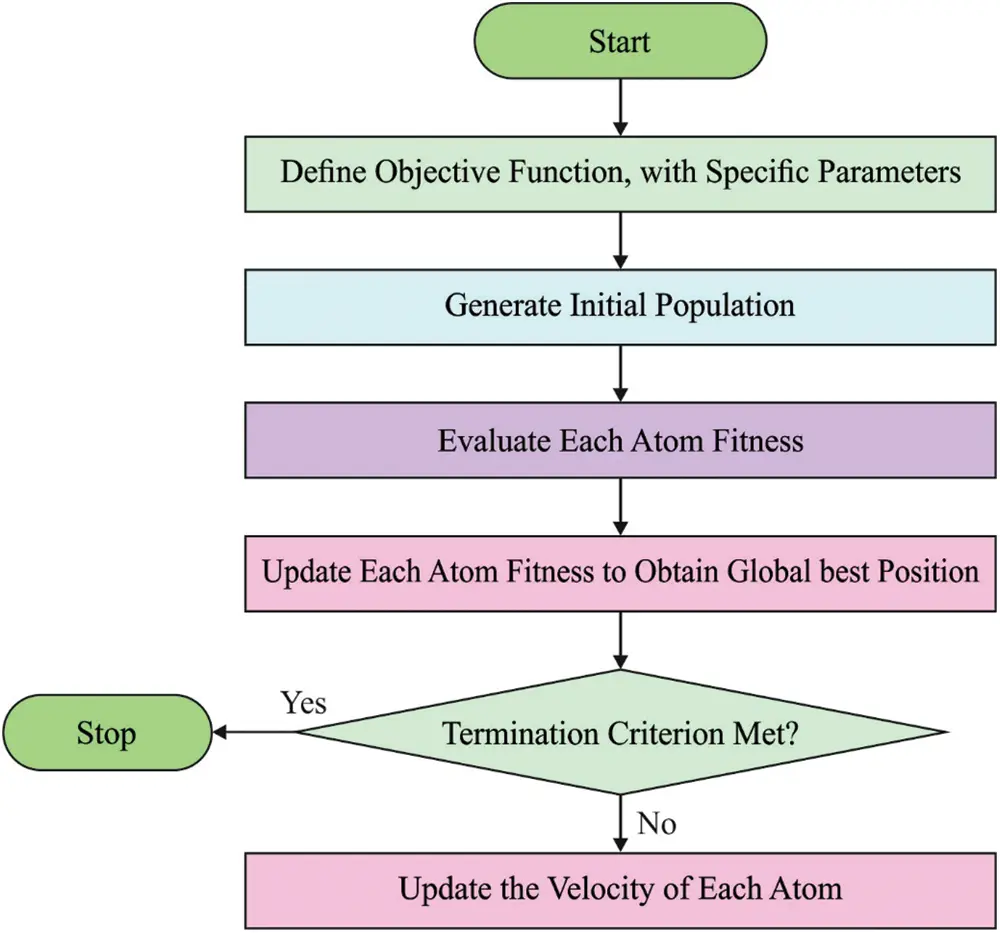Figure 2: Flowchart of ASO technique

Mi(t)=eFiti(t)Fitbest(t)Fitworst(t)Fitbest(t)(13)

mi(t)=Mi(t)j=1NMj(t)(14)

Now Fiti(t) indicates the FF of i-th atom in t iteration, Fitbest(t) and Fitworst(t) denote the fitness values of optimal and the worst atoms during t iteration, correspondingly

Fitbest(t)=miniϵ{1,2,N}Fiti(t)(15)

Fitworst(t)=maxiϵ{1,2,N}Fiti(t)(16)

At last, the position and velocity of i-th atom are updated during (t+1) iteration and is described as follows.

vid(t+1)=randidvid(t)+aid(t)(17)

xid(t+1)=xid(t)+vid(t+1)(18)

2.2 Processes Involved in ASO-Based Clustering Technique

The presented ASO model derives a FF that involves multiple parameters especially trust parameters for secure process. The RE of UAV (x), when transferring k bits to the terminus UAV (y), on distance d, is determined as follows.

RE=E(ET(k,d)+ER(k))(19)

In Eq. (19), E indicates the present energy of UAV and ET denotes the energy expended for sensing the information.

In Eq. (20), Ee represents the energy of electrons and Ea denotes the amplified energy, ER(k) signifies the energy dissipated to obtain information as given below.

ER(k)=kEe(21)

Three variables are used to select the CH and calculate the average distance (AvgD) for neighboring UAVs. Here, AvgD symbolizes the average distance value to the UAV and its individual neighbouring UAV as given below.

AvgNBDisti=j=1NBidist(i,nbj)NBi,(22)

In Eq. (22), dist(i,nbj) denotes the distance from UAV to the neighbouring jth UAV.

Here, energy trust is measured if the node has sufficient RE for completing novel transmissions and data processing tasks. The present research case made use of direct trust between the nodes, A as well as B of all the clusters, and their mathematical model is signified as follows.

At this point, Trijdir and TRijindir represent the direct as well as indirect trust values of one node to another node correspondingly.

2.3 Signcryption Process

In this work, signcryption technique is applied to effectually encrypt the images captured by drones in IoD environment which enables secure transmission of the images to ground station . Here, public key encryption system is used as a security approach in which digital signature is used and it optimizes integrity, authenticity, confidentiality, availability, and nonrepudiation. Encryption can be done instead of modest encryption. Further, single session keys are also reprocessed for some encryption to obtain remarkable outcome than signcryption system. Essentially, signcryption methodology has the following processes namely, designcryption stage, key generation, and signcryption process. Meanwhile, encryption offers security and signature provides authenticity. In parallel, signcryption implements both encryption and signature functions from the logical stage itself. However, the overhead values of communication and calculation are less than the series of signatures. In signcryption process, there exists different stages such as key generation, initialization of parameters, designcryption, and signcryption. Initially, signature-based security analysis allows the variables namely huge prime values for sender and receiver keys, key generation, and hash values.

In this method, the keys are produced with the help of cryptographic technique; the function of the elliptical curve-based shape is to generate the prime numbers and influence the numbers created. The initializing parameters are Sr1, Su1, Rr2, and Ru1. In order to maximize the secure communication, the key is utilized.

i)   Encryption technique transfers the dataset to receiver after analyzing the security; both hash and one-keyed hash values are taken into account based on the encrypted data as well as motion vector. This transformation of plain dataset into ciphered dataset can be defined in subsequent phases. At first, the sender transmits the data with a suitable value, A from [1,,PF---1].

ii)   The hash values of the sender are evaluated using Ru2 receiver and A denotes the output OHo of hash values i.e., 128bits. The mathematical expression of the equation is given below.

OHo=HASH(Ru2AmodPN).(23)

iii)   The output values of 128bits are partitioned into 64 pieces with each piece containing two bits such as OHo1 and OHo2.

iv)   The sender encrypts the data for E encryption as well as OHo1. It is defined as follows.

Ci=EOHo1(info).(24)

v)   Next, the OHo2 values are efficiently employed from one-way keyed hash function KHo to hash the data that results in 128bits hash which is characterized as follows.

F=KHo2(info).(25)

vi)   At last, the signcryption of the data is estimated. Then, the cipher dataset is characterized as follows.

S=A/(F+AOHO1)modPF(26)

vii)   From the estimation, three different values, F, and Ci are transferred to the receiver and sender.

3  Experimental Validation

The current section validates the clustering and encryption performance of the proposed ASOCE-SIoD model under diverse number of drones and grid sizes. A comparison study was conducted with existing models such as Ant Colony Optimization (ACO), Grey Wolf Optimizer (GWO), and blockchain with clustering in IoD (BICIoD).

Tab. 1 provides a detailed overview on Cluster Building Time (CBT) analysis results achieved by ASOCE-SIoD model and other existing models on grids sized such as (1000 × 1000 m) and (2000 × 2000 m). Fig. 3 exhibits the results of comparative CBT analysis accomplished by ASOCE-SIoD model and other existing models under the grid sized at 1000 × 1000 m and distinct number of drones. The figure indicates that the proposed ASOCE-SIoD model required less CBT over existing techniques. For instance, with 15 drones, the proposed ASOCE-SIoD model required the least CBT of 1.73 s, whereas ACO, GWO, and BICIoD techniques demanded high CBT such as 6.04, 4.75, and 2.81 s respectively. Along with that, with 35 drones, the proposed ASOCE-SIoD approach required a minimal CBT of 3.24 s, whereas ACO, GWO, and BICIoD methods obtained the maximal CBT of 23.52, 14.03, and 4.75 s correspondingly.Figure 3: CBT analysis results of ASOCE-SIoD technique under grid size, 1000 × 1000 m

Fig. 4 demonstrates the comparative CBT examination results achieved by the proposed ASOCE-SIoD methodology and other techniques under grid size 2000 × 2000 m and distinct number of drones. The figure expose that the proposed ASOCE-SIoD system required less CBT over existing approaches. For sample, with 15 drones, the proposed ASOCE-SIoD algorithm required the least CBT of 2.05 s, whereas ACO, GWO, and BICIoD systems demanded the maximum CBT such as 8.19, 6.62, and 4.57 s correspondingly. Likewise, with 35 drones, ASOCE-SIoD system required the least CBT of 5.20 s, whereas ACO, GWO, and BICIoD techniques demanded high CBT such as 27.56, 13.54, and 8.58 s correspondingly. Tab. 2 provides a detailed overview on Energy Consumption (ECM) analysis results accomplished by the proposed ASOCE-SIoD methodology and other approaches on grids sized at (1000 × 1000 m) and (2000 × 2000 m).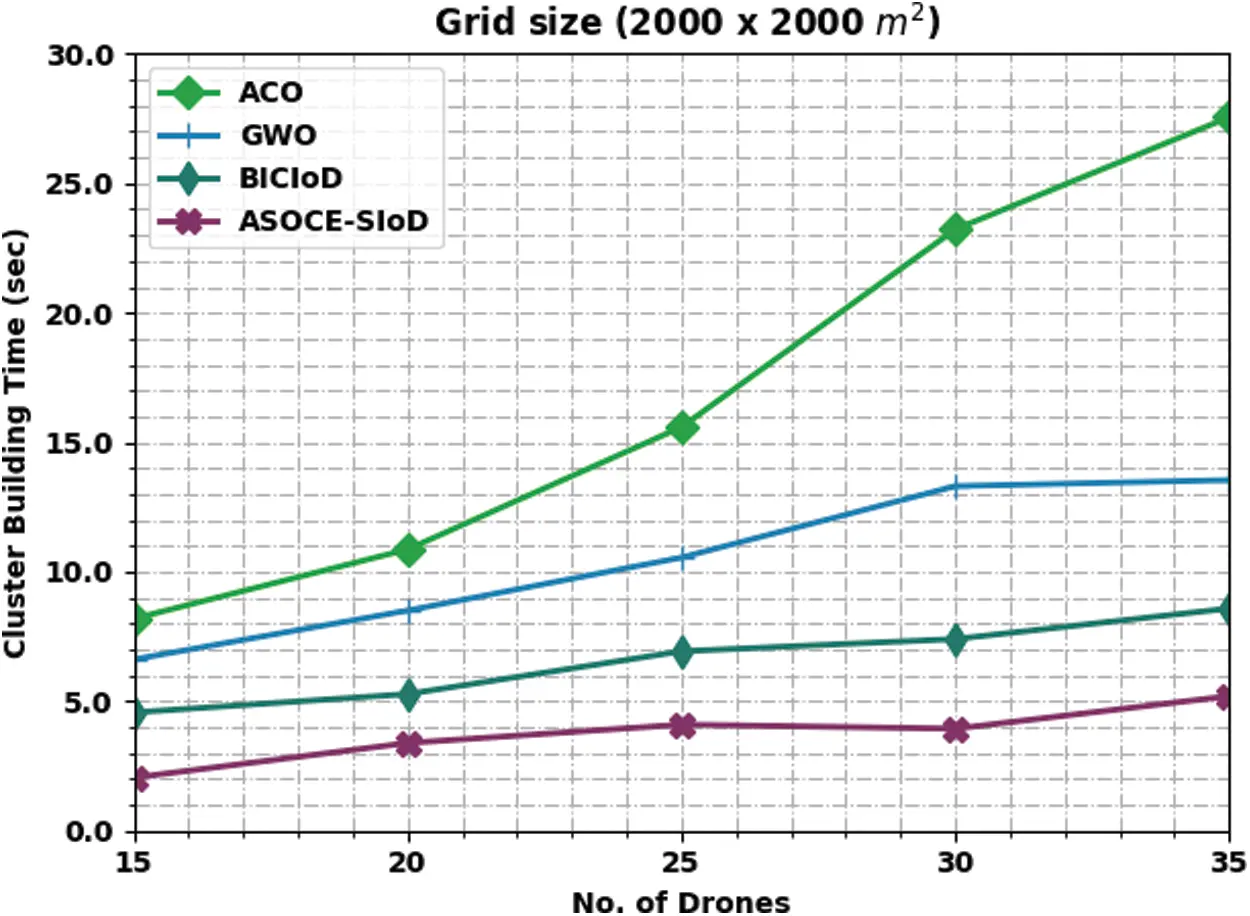Figure 4: CBT analysis results of ASOCE-SIoD technique under grid size, 2000 × 2000 mFig. 5 showcases the comparative Energy Consumption (ECM) analysis results accomplished by the proposed ASOCE-SIoD methodology and other existing approaches under grid size of 1000 × 1000 m and distinct number of drones. The figure shows the superior performance of ASOCE-SIoD system with low ECM over existing approaches. For sample, with 15 drones, the proposed ASOCE-SIoD methodology obtained the ECM of 0.56 J, whereas ACO, GWO, and BICIoD techniques obtained high ECM values such as 1.73, 1.94, and 1.24 J respectively. Eventually, with 35 drones, the proposed ASOCE-SIoD technique obtained the least ECM of 2.25 J, whereas ACO, GWO, and BICIoD algorithms obtained high ECM values such as 4.40, 5.13, and 3.20 J correspondingly.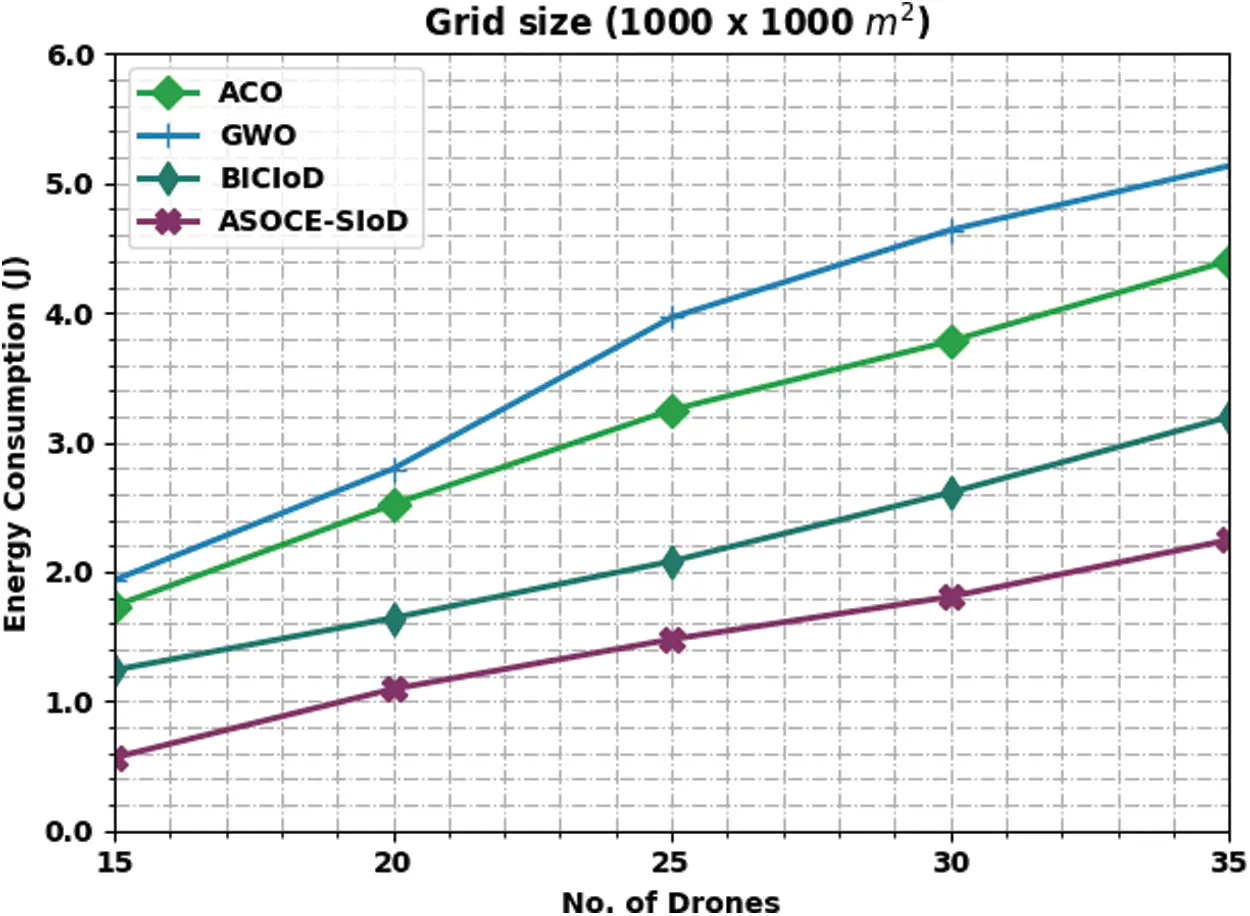Figure 5: ECM analysis results of ASOCE-SIoD algorithm under grid size, 100 × 1000 m

Fig. 6 portrays the comparative ECM inspection results achieved by the proposed ASOCE-SIoD model and other existing methods under grid size of 2000 × 2000 m and distinct number of drones. The figure expose that the proposed ASOCE-SIoD system achieved a low ECM over existing techniques. For instance, with 15 drones, ASOCE-SIoD model offered the least ECM of 0.92 J, whereas ACO, GWO, and BICIoD techniques obtained high ECM values such as 2.19, 2.52, and 1.52 J correspondingly. In addition, with 35 drones, the proposed ASOCE-SIoD approach achieved the least ECM of 2.98 J, whereas ACO, GWO, and BICIoD systems gained enhanced ECM values such as 4.71, 5.26, and 4.13 J correspondingly.Figure 6: ECM analysis results of ASOCE-SIoD algorithm under grid size 2000 × 2000 m

A detailed Cluster Lifetime (CLT) analysis was conducted between ASOCE-SIoD model and other existing approaches and the results are shown in Tab. 3 under distinct sizes of grids and drones.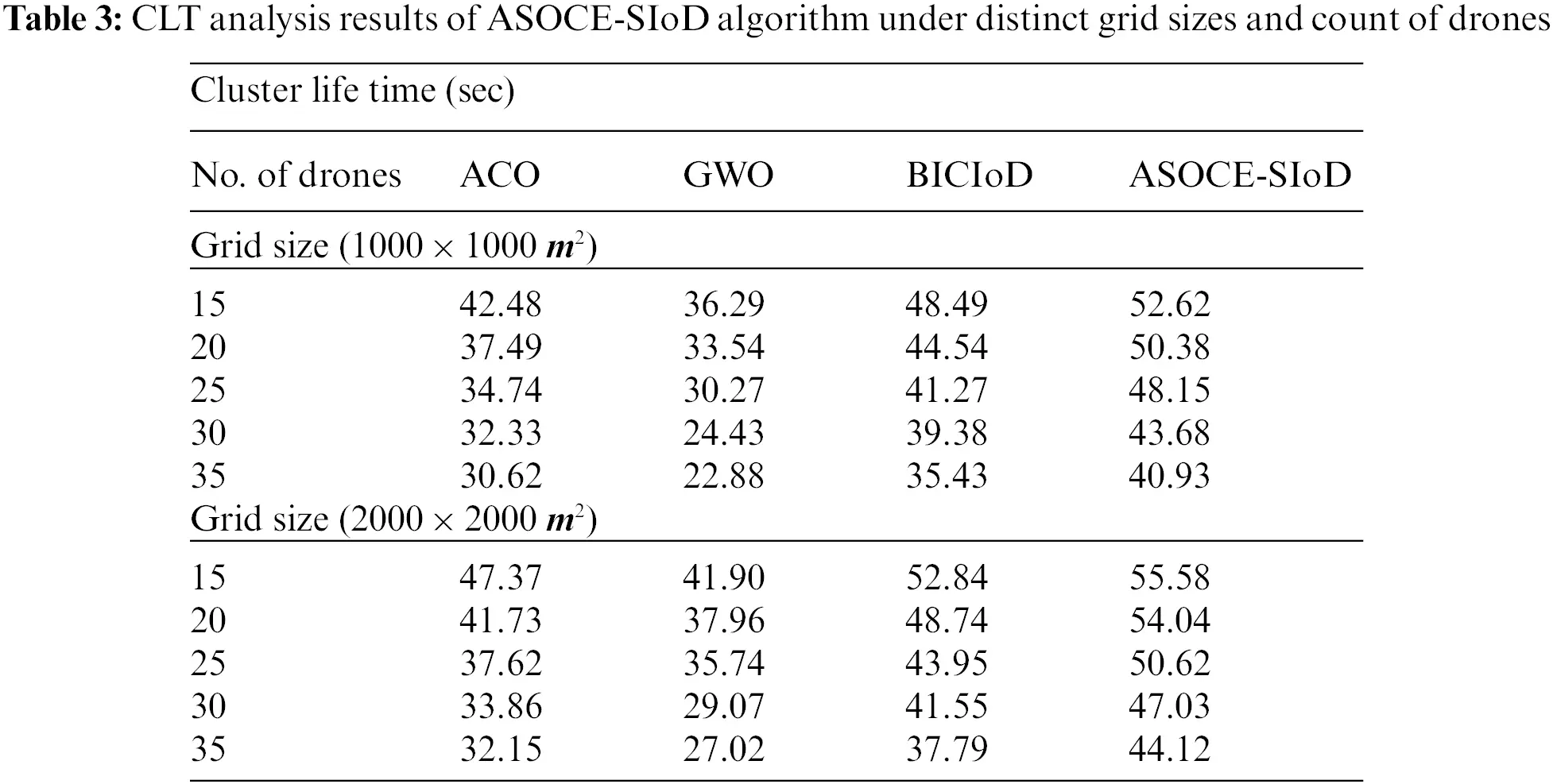Fig. 7 exhibits the comparative CLT results accomplished by the proposed ASOCE-SIoD system and other existing models on grid size of 1000 × 1000 m. The figure implies that the proposed ASOCE-SIoD approach demonstrated effectual outcomes with increased CLT values under all the cases. For instance, with 15 drones, ASOCE-SIoD model attained a high CLT of 52.62 s, whereas ACO, GWO, and BICIoD techniques reached the least CLT values such as 42.48, 36.29, and 48.49 s respectively. Also, with 35 drones, the proposed ASOCE-SIoD system obtained a superior CLT of 40.93 s, whereas ACO, GWO, and BICIoD techniques attained minimal CLT values such as 30.62, 22.88, and 35.43 s correspondingly.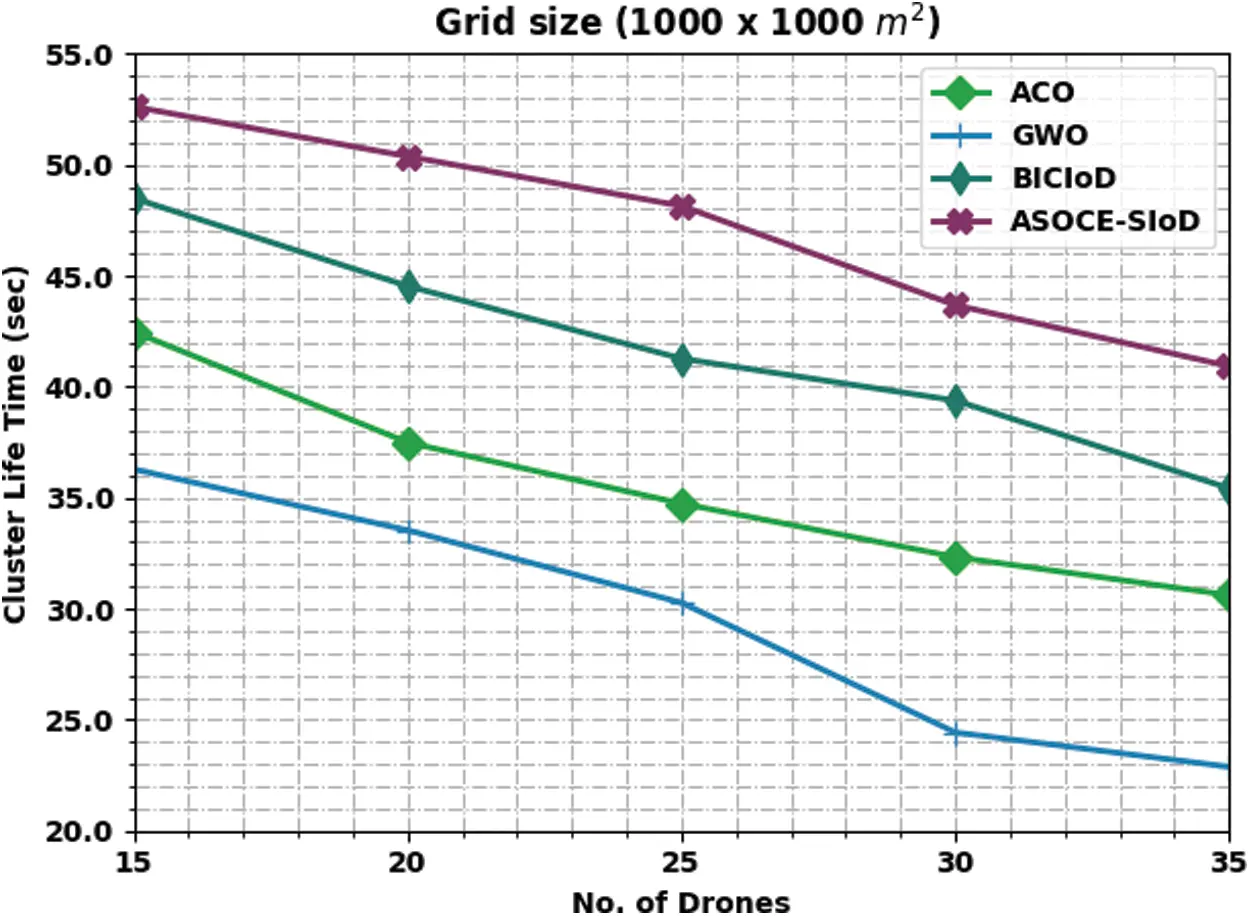Figure 7: CLT analysis results of ASOCE-SIoD technique under grid size of 1000 × 1000 m

Fig. 8 shows the comparative CLT results attained by the proposed ASOCE-SIoD model with other existing approaches on grid size of 2000 × 2000 m. The figure infers that the proposed ASOCE-SIoD system depicted effectual outcomes with high CLT values under all the cases. For instance, with 15 drones, the proposed ASOCE-SIoD model obtained the maximum CLT of 55.58 s, whereas ACO, GWO, and BICIoD techniques reached low CLT values such as 47.37, 41.90, and 52.84 s correspondingly. Furthermore, with 35 drones, ASOCE-SIoD algorithm attained a high CLT of 44.12 s, whereas ACO, GWO, and BICIoD systems reached low CLT values such as 32.15, 27.02, and 37.79 s correspondingly.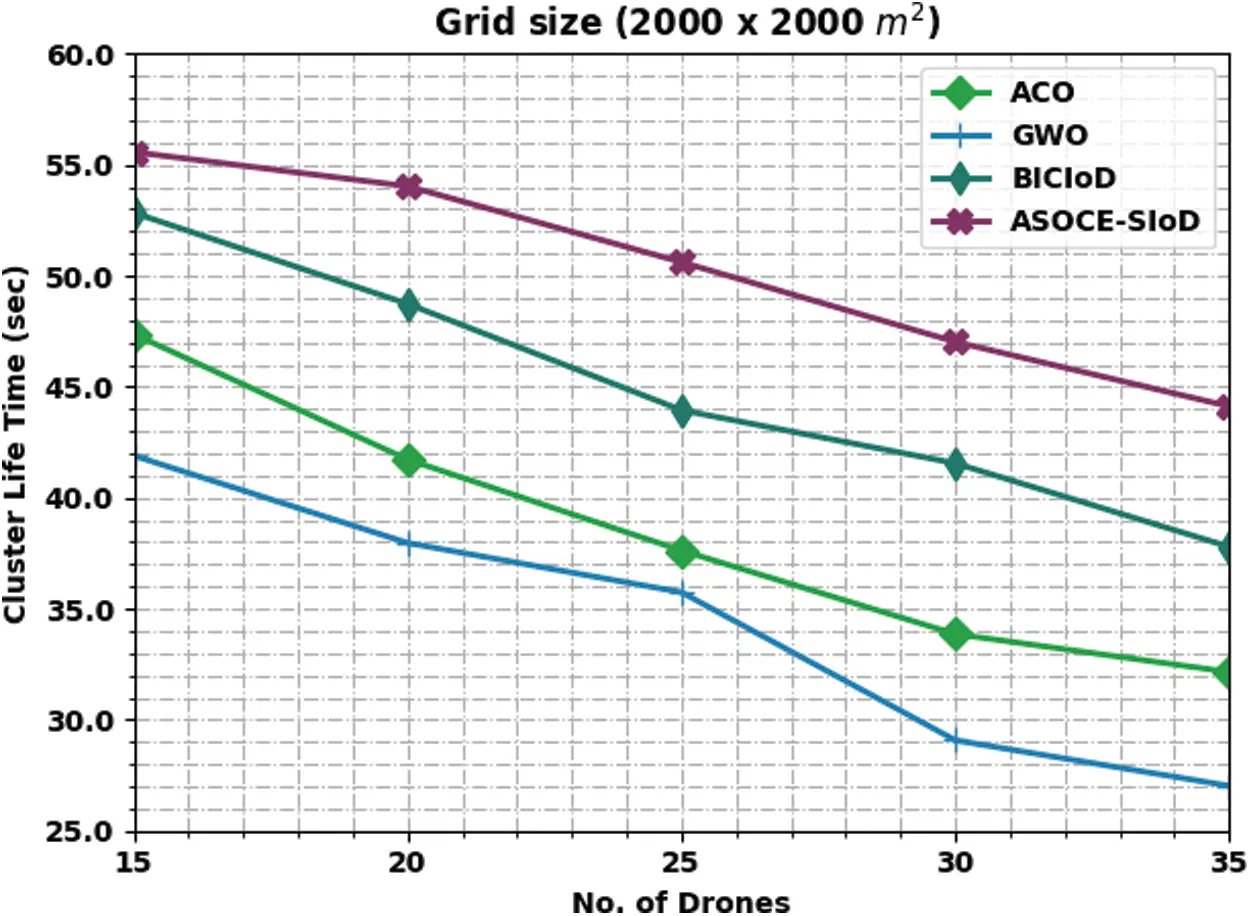Figure 8: CLT analysis results of ASOCE-SIoD technique under grid size of 2000 × 2000 m

Tab. 4 reports the overall analysis results achieved by the proposed ASOCE-SIoD model under different measures such as Mean Square Error (MSE), Peak Signal to Noise Ratio (PSNR), and Correlation Coefficient (CC). The results indicate that the proposed ASOCE-SIoD model offered low MSE values and high PSNR values and CC on all the images. For instance, with image 1, the proposed ASOCE-SIoD model provided an MSE of 0.071, PSNR of 59.618 dB, and a CC of 99.52. Also, with image 3, ASOCE-SIoD method yielded an MSE of 0.085, PSNR of 58.837 dB, and a CC of 99.49. At the same time, with image 5, the proposed ASOCE-SIoD algorithm offered an MSE of 0.126, PSNR of 57.127 dB, and a CC of 99.77.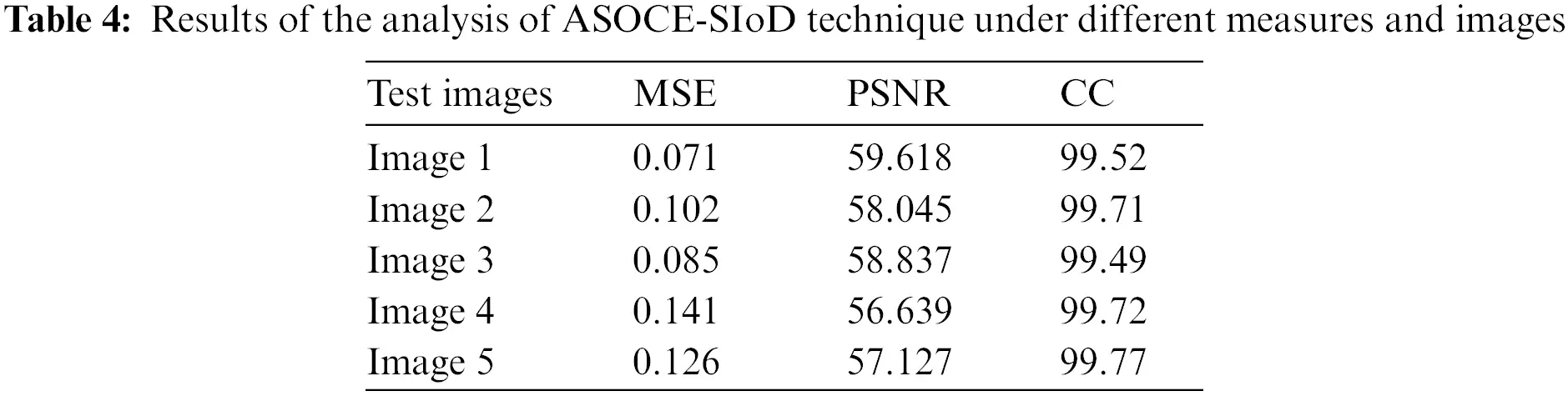Tab. 5 provides the comparative MSE and PSNR analysis results yielded by the proposed ASOCE-SIoD model. Fig. 9 briefly illustrates the MSE inspection results attained by ASOCE-SIoD model with other models. The results imply that ASOCE-SIoD model achieved less MSE values for all the images. For image 1, ASOCE-SIoD approach resulted in less MSE of 0.071, whereas Share Creation (SC) scheme with Social Spider Optimization (SSO)-based Optimal Elliptic Curve Cryptography (ECC) called SC-SSOECC, HMOA-ECC, and Particle Swarm Optimization-based ECC (PSO-ECC) approaches gained high MSE values such as 0.094, 0.175, and 0.287 correspondingly. Besides, for image5, ASOCE-SIoD model provided the least MSE of 0.126, whereas SC-SSOECC, HMOA-ECC, and PSO-ECC approaches achieved high MSE values such as 0.177, 0.184, and 0.232 correspondingly.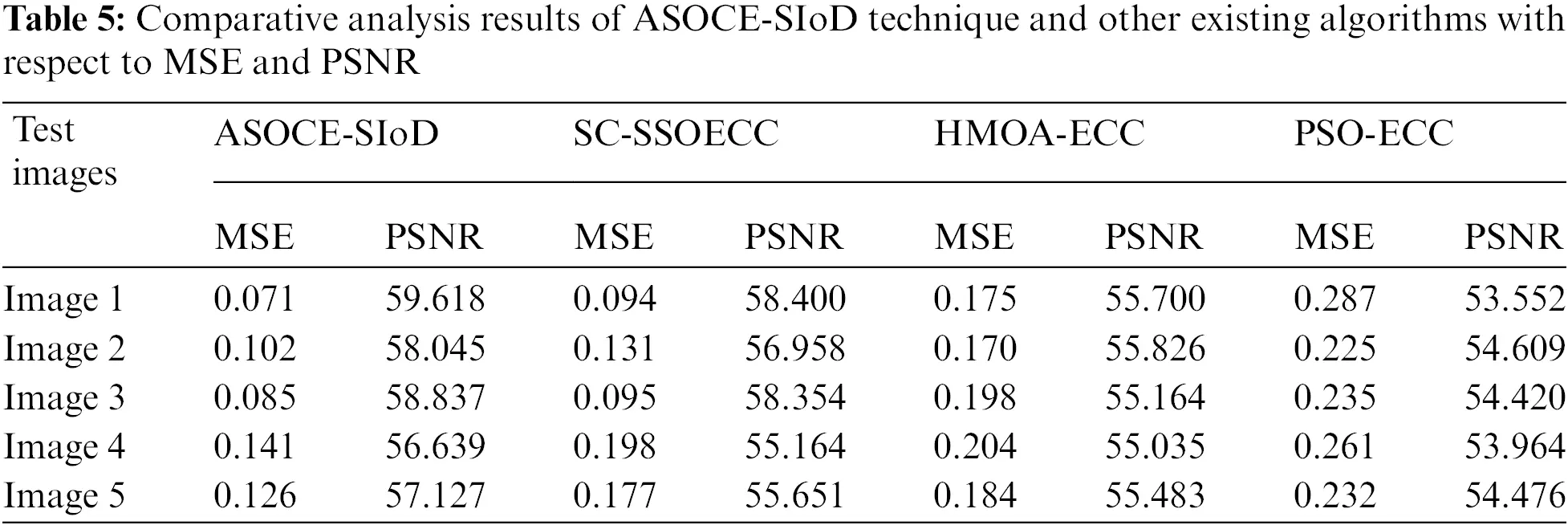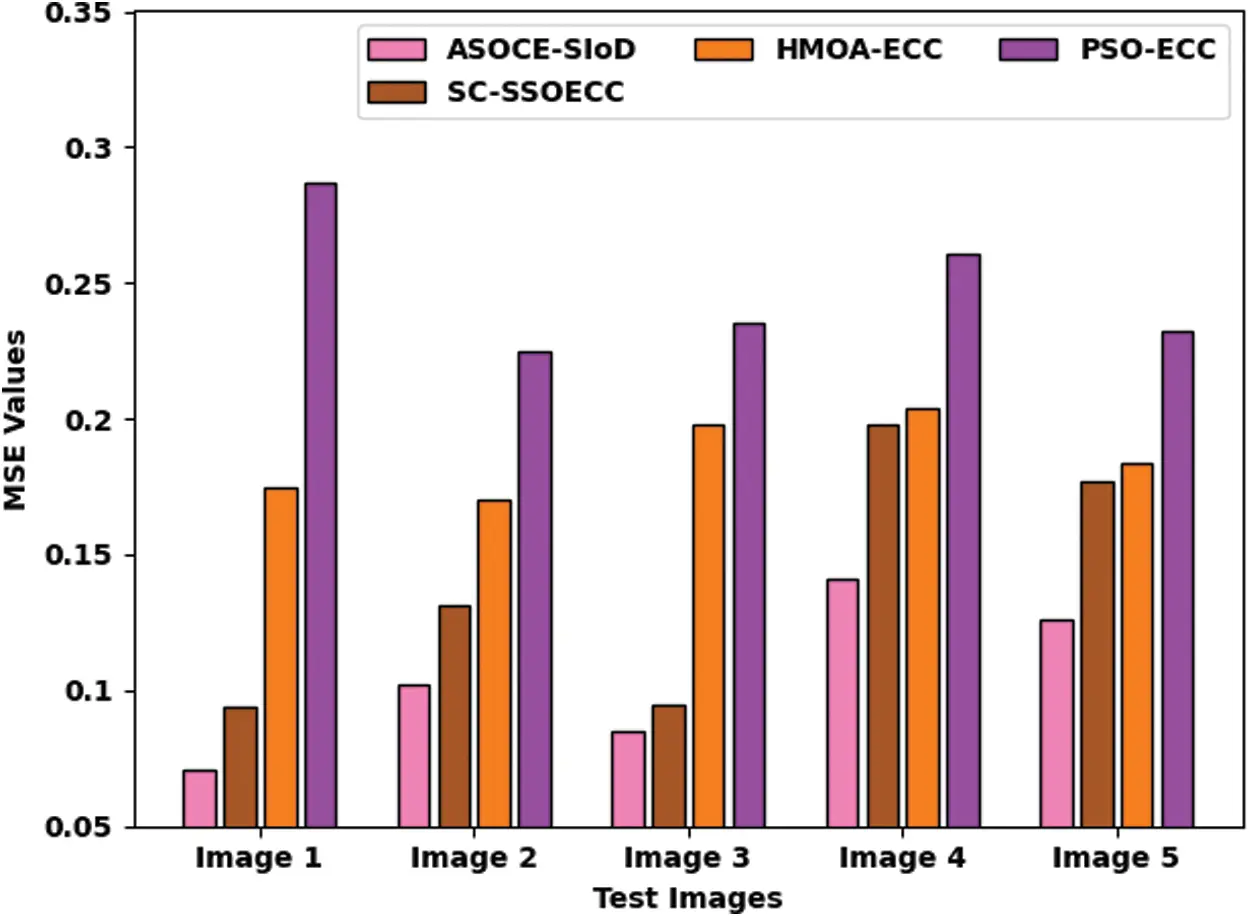Figure 9: MSE analysis results of ASOCE-SIoD algorithm with recent methodologies

A detailed PSNR analysis was conducted between the proposed ASOCE-SIoD technique and other existing algorithms on distinct images and the results are shown in Fig. 10. The outcomes expose that the proposed ASOCE-SIoD system demonstrated high PSNR values. For image1, ASOCE-SIoD approach gained the maximum PSNR value of 59.618 dB, while SC-SSOECC, HMOA-ECC, and PSO-ECC algorithms reached minimal PSNR values such as 58.400, 55.700, and 53.552 dB respectively. Along with that, for image5, the proposed ASOCE-SIoD approach obtained the maximum PSNR of 57.127 dB, whereas SC-SSOECC, HMOA-ECC, and PSO-ECC algorithms gained the least PSNR values such as 55.651, 55.483, and 54.476 dB correspondingly. Based on the results and discussion made above, it is evident that the proposed ASOCE-SIoD model is superior to ther techniques.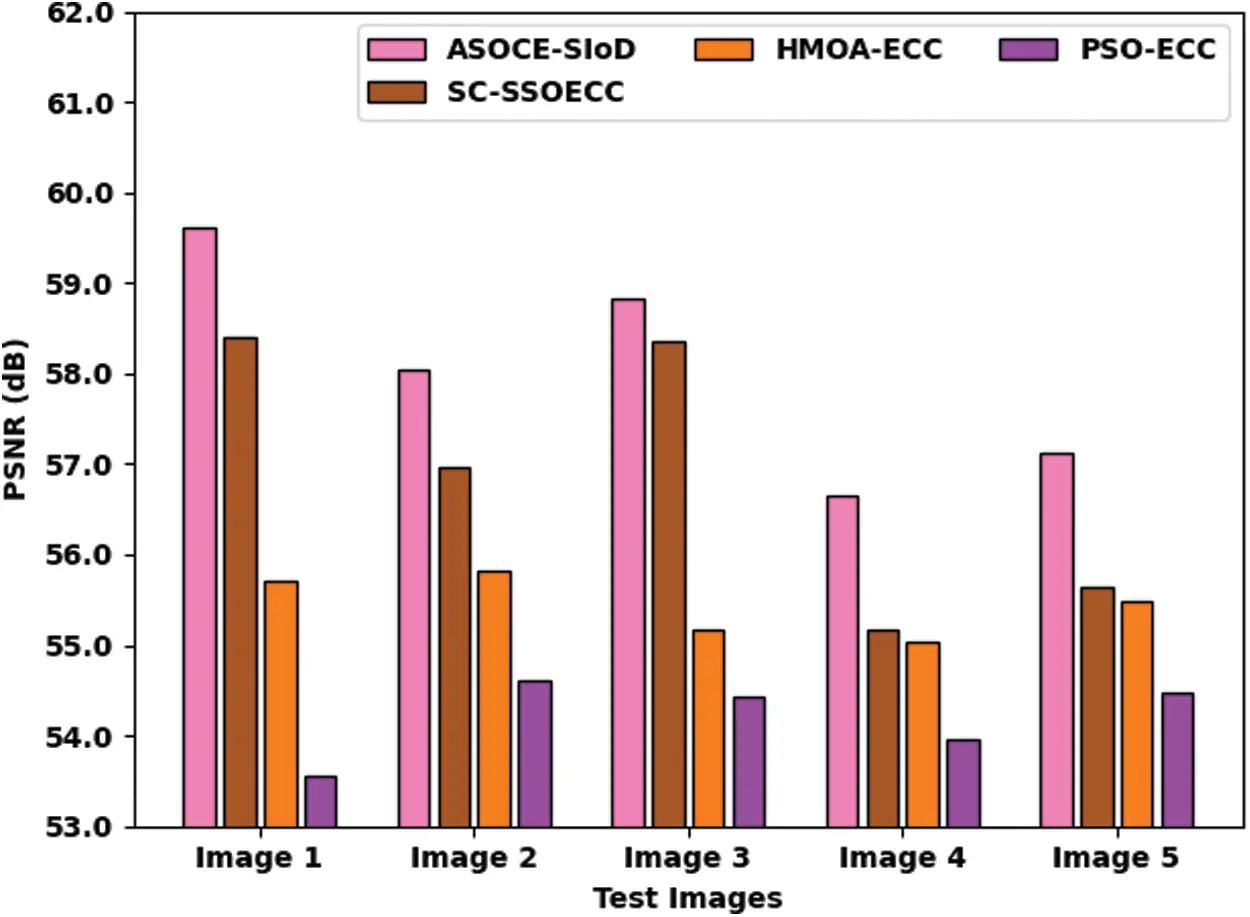Figure 10: PSNR analysis results of ASOCE-SIoD algorithm with existing algorithms

4  Conclusion

In this study, a novel ASOCE-SIoD approach has been developed to group the drones into clusters and encrypt the images captured by drones. The presented ASOCE-SIoD technique follows ASO-based CH and cluster construction technique. The presented model derives an FF involving multiple parameters especially trust parameters for secure process. In addition, signcryption technique is also applied to effectually encrypt the images captured by drones in IoD environment which enables the secure transmission of images to ground station. In order to validate the better performance of the proposed ASOCE-SIoD model, different experimental analyses were conducted and the outcomes were examined under different aspects. A comprehensive comparison study was conducted and the results highlighted the betterment of ASOCE-SIoD model over recent approaches. In future, lightweight cryptographic solutions can be derived to increase the security levels in IoD environment.

Funding Statement: The authors extend their appreciation to the Deanship of Scientific Research at King Khalid University for funding this work through Large Groups Project Under Grant Number (46/43). Princess Nourah bint Abdulrahman University Researchers Supporting Project Number (PNURSP2022R238), Princess Nourah bint Abdulrahman University, Riyadh, Saudi Arabia. The authors would like to thank the Deanship of Scientific Research at Umm Al-Qura University for supporting this work by Grant Code: 22UQU4340237DSR25.

Conflicts of Interest: The authors declare that they have no conflicts of interest to report regarding the present study.

## References

1. L. Abualigah, A. Diabat, P. Sumari and A. H. Gandomi, “Applications, deployments, and integration of internet of drones (iodA review,” IEEE Sensors Journal, vol. 21, no. 22, pp. 25532–25546, 202
2. M. Yahuza, M. Y. I. Idris, I. B. Ahmedy, A. W. A. Wahab, T. Nandy et al., “Internet of drones security and privacy issues: Taxonomy and open challenges,” IEEE Access, vol. 9, pp. 57243–57270, 2021.
3. Y. Zhang, D. He, L. Li and B. Chen, “A lightweight authentication and key agreement scheme for internet of drones,” Computer Communications, vol. 154, pp. 455–464, 2020.
4. C. Lin, D. He, N. Kumar, K. K. R. Choo, A. Vinel et al., “Security and privacy for the internet of drones: Challenges and solutions,” IEEE Communications Magazine, vol. 56, no. 1, pp. 64–69, 2018.
5. Y. Tian, J. Yuan and H. Song, “Efficient privacy-preserving authentication framework for edge-assisted internet of drones,” Journal of Information Security and Applications, vol. 48, pp. 102354, 2019.
6. G. Choudhary, V. Sharma, T. Gupta, J. Kim and I. You, “Internet of drones (IoDThreats, vulnerability, and security perspectives,” arXiv preprint arXiv:1808.00203, 2018.
7. S. A. Chaudhry, K. Yahya, M. Karuppiah, R. Kharel, A. K. Bashir et al., “GCACS-IoD: A certificate based generic access control scheme for internet of drones,” Computer Networks, vol. 191, pp. 107999, 2021.
8. F. Aftab, A. Khan and Z. Zhang, “Hybrid self-organized clustering scheme for drone based cognitive internet of things,” IEEE Access, vol. 7, pp. 56217–56227, 2019.
9. M. N. A. Mhiqani, R. Ahmad, Z. Z. Abidin, K. H. Abdulkareem, M. A. Mohammed et al., “A new intelligent multilayer framework for insider threat detection,” Computers & Electrical Engineering, vol. 97, pp. 107597, 2022.
10. O. A. Alzubi, J. A. Alzubi, K. Shankar and D. Gupta, “Blockchain and artificial intelligence enabled privacy-preserving medical data transmission in internet of things,” Transactions on Emerging Telecommunications Technologies, vol. 32, no. 12, 2021.
11. B. Bera, D. Chattaraj and A. K. Das, “Designing secure blockchain-based access control scheme in IoT-enabled internet of drones deployment,” Computer Communications, vol. 153, pp. 229–249, 2020.
12. M. Wazid, A. K. Das, N. Kumar, A. V. Vasilakos and J. J. P. C. Rodrigues, “Design and analysis of secure lightweight remote user authentication and key agreement scheme in internet of drones deployment,” IEEE Internet Things Journal, vol. 6, no. 2, pp. 3572–3584, 2019.
13. F. Aftab, A. Khan and Z. Zhang, “Bio-inspired clustering scheme for internet of drones application in industrial wireless sensor network,” International Journal of Distributed Sensor Networks, vol. 15, no. 11, pp. 155014771988990, 2019.
14. A. Saif, K. Dimyati, K. A. Noordin, N. S. M. Shah, S. H. Alsamhi et al., “Distributed clustering for user devices under uav coverage area during disaster recovery,” in 2021 IEEE Int. Conf. in Power Engineering Application (ICPEA), Malaysia, pp. 143–148, 2021.
15. F. Alrowais, A. S. Almasoud, R. Marzouk, F. N. Al-Wesabi, A. M. Hilal et al., “Artificial intelligence-based data offloading technique for secure MEC systems,” Computers, Materials & Continua, vol. 72, no. 2, pp. 2783–2795, 2022.
16. A. M. Hilal, J. S. Alzahrani, I. Abunadi, N. Nemri, F. N. Al-Wesabi et al., “Intelligent deep learning model for privacy preserving IIoT on 6g environment,” Computers, Materials & Continua, vol. 72, no. 1, pp. 333–348, 2022.
17. I. Abunadi, M. M. Althobaiti, F. N. Al-Wesabi, A. M. Hilal, M. Medani et al., “Federated learning with blockchain assisted image classification for clustered uav networks,” Computers, Materials & Continua, vol. 72, no. 1, pp. 1195–1212, 2022.
18. B. Bera, S. Saha, A. K. Das, N. Kumar, P. Lorenz et al., “Blockchain-envisioned secure data delivery and collection scheme for 5g-based iot-enabled internet of drones environment,” IEEE Transactions on Vehicular Technology, vol. 69, no. 8, pp. 9097–9111, 2020.
19. B. Hekimoglu, “Optimal tuning of fractional order pid controller for dc motor speed control via chaotic atom search optimization algorithm,” IEEE Access, vol. 7, pp. 38100–38114, 20
20. W. Zhao, L. Wang and Z. Zhang, “Atom search optimization and its application to solve a hydrogeologic parameter estimation problem,” Knowledge-Based Systems, vol. 163, pp. 283–304, 2019.
21. M. Elhoseny and K. Shankar, “Reliable data transmission model for mobile ad hoc network using signcryption technique,” IEEE Transactions on Reliability, vol. 69, no. 3, pp. 1077–1086, 2020.This work is licensed under a Creative Commons Attribution 4.0 International License, which permits unrestricted use, distribution, and reproduction in any medium, provided the original work is properly cited.